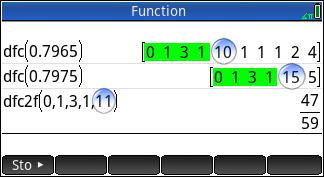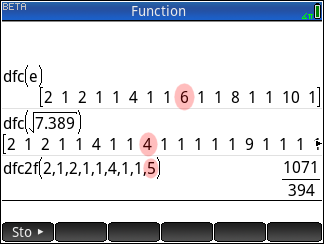The new dfc and dfc2f functions in action
01-10-2018, 01:37 AM (This post was last modified: 01-10-2018 01:48 AM by Joe Horn.)
Post: #1Joe HornSenior Member Posts: 1,920 Joined: Dec 2013
The new dfc and dfc2f functions in action
A whole class of difficult-to-solve problems has been rendered easy to solve due to the addition of two new functions to the HP Prime (dfc and dfc2f) in a recent firmware upgrade. Here's one simple example of such a problem, and how to solve it using dfc and dfc2f.

PROBLEM: What is the simplest fraction which evaluates to 0.797 when rounded to 3 decimal places? In other words, what's the simplest fraction in the half-open interval [0.7965, 0.7975)?

The answer can be found in these 3 easy steps:Here's a step-by-step explanation of what happened above. The first two lines are both ends of the desired interval being fed into the dfc() function. Take careful note of where the two outputs begin to differ. In this case, the first 4 elements are the same (0,1,3,1), but after that they begin to differ (one is 10 and the other is 15). All you need to do is take the smaller one (10), add one to it (11), and drop all the remaining elements, and feed that new list (0,1,3,1,11) into the dfc2f function to obtain the final answer (47/59). Easy peasy.

Warning: Since the interval is half open, make sure your answer is not the right endpoint. If it is, adjust the last number in the dfc2f list by 1. If that yields the left endpoint, then that's the best possible answer. (This probably only happens in pathological cases which are invented to make algorithms choke, but that's what I do for fun, so that's how to handle it.)

Writing a program which automates this process is left as an exercise for the student.EDIT: Warning: If you use the dfc2f function in Home, and it returns a real, not a fraction, then press Shift CAS (Settings) and turn on the "Change apparent integers into exact integers" setting, which is the checkbox at the end of the 3rd row. Then it'll return a fraction in Home, as it always does in CAS.

EDIT 2: Homework: What's the simplest fraction between the constant $$e$$ and $$\sqrt { 7.389 }$$ ?

<0|ɸ|0>
-Joe-
01-10-2018, 07:47 AM
Post: #2salvomicSenior Member Posts: 1,396 Joined: Jan 2015
RE: The new dfc and dfc2f functions in action
Is it 1457/536?
this is
2.718283582209
e^1 is
2.71828182846
√7.389 is
2.71827150962
so the equal digits are:
2.7182, then there is 8 or 7, so we have 4 digit to round...

Am I wrong?Salvo

Attached File(s) Thumbnail(s)∫aL√0mic (IT9CLU) :: HP Prime 50g 41CX 71b 42s 39s 35s 12C 15C - DM42, DM41X - WP34s Prime Soft. Lib
01-10-2018, 02:07 PM (This post was last modified: 01-10-2018 02:09 PM by DrD.)
Post: #3
 DrDSenior Member Posts: 1,133 Joined: Feb 2014
RE: The new dfc and dfc2f functions in action
I tried this:

Code:
 #cas edfc(nbr1:=e,nbr2:=sqrt(7.389)):= begin     LOCAL n;   M0:=dfc(nbr1);   M1:=dfc(nbr2);   M2:=MAX(M0,M1)-MIN(M0,M1);   n:=POS(mat2list(map(M2,(x)->x>0)),1);  // Probably a better way to find a non-zero element in vector?   L1:=sub(M0,1,n);   L2:=sub(M1,1,n);    IF L1(n)<=L2(n) THEN     L1(n):=L1(n)+1;     n:=dfc2f(L1);   ELSE     L2(n):=L2(n)+1;     n:=dfc2f(L2);    END;   return n; end; #end

edfc(); // uses defaults: [ e,sqrt(7.389) ) ==> 1071/394 = 2.71827411168

edfc(0.7965, 0.7975); // From first example: ==> 47/59 = 0.796610169492

This is just a quick program push out, not completely tested!!

-Dale-
01-11-2018, 02:33 AM
Post: #4Joe HornSenior Member Posts: 1,920 Joined: Dec 2013
RE: The new dfc and dfc2f functions in action
(01-10-2018 07:47 AM)salvomic Wrote:  Is it 1457/536?

No. You're supposed to take the SMALLER of the two first-differing numbers in the dfc output (in this case, 4) and add 1 to that. Like this:It seems that you may have been looking for where the decimal expansions of the inputs begin to differ. Don't do that. Look instead at the outputs of dfc and see where THOSE begin to differ. The entire algorithm is illustrated in the above screen shot. The decimal expansions have nothing to do with it.

<0|ɸ|0>
-Joe-
01-11-2018, 05:55 AM
Post: #5
 webmasterpdxSenior Member Posts: 541 Joined: Sep 2015
RE: The new dfc and dfc2f functions in action
Continued Fraction section has been added to the Prime wiki under Programming Documentation.
http://www.wiki4hp.com/doku.php?id=prime:start
01-11-2018, 07:23 AM (This post was last modified: 01-11-2018 07:27 AM by salvomic.)
Post: #6salvomicSenior Member Posts: 1,396 Joined: Jan 2015
RE: The new dfc and dfc2f functions in action
(01-11-2018 02:33 AM)Joe Horn Wrote:  It seems that you may have been looking for where the decimal expansions of the inputs begin to differ. Don't do that. Look instead at the outputs of dfc and see where THOSE begin to differ. The entire algorithm is illustrated in the above screen shot. The decimal expansions have nothing to do with it.

Well, thank you. Understood now.
I confronted 6 and 4, but increased 6 to 7 and not 4 to 5...

Salvo

∫aL√0mic (IT9CLU) :: HP Prime 50g 41CX 71b 42s 39s 35s 12C 15C - DM42, DM41X - WP34s Prime Soft. Lib
07-10-2018, 06:47 AM
Post: #7Joe HornSenior Member Posts: 1,920 Joined: Dec 2013
RE: The new dfc and dfc2f functions in action
(01-10-2018 02:07 PM)DrD Wrote:  I tried this:

Code:
 #cas edfc(nbr1:=e,nbr2:=sqrt(7.389)):= begin     LOCAL n;   M0:=dfc(nbr1);   M1:=dfc(nbr2);   M2:=MAX(M0,M1)-MIN(M0,M1);   n:=POS(mat2list(map(M2,(x)->x>0)),1);  // Probably a better way to find a non-zero element in vector?   L1:=sub(M0,1,n);   L2:=sub(M1,1,n);    IF L1(n)<=L2(n) THEN     L1(n):=L1(n)+1;     n:=dfc2f(L1);   ELSE     L2(n):=L2(n)+1;     n:=dfc2f(L2);    END;   return n; end; #end

edfc(); // uses defaults: [ e,sqrt(7.389) ) ==> 1071/394 = 2.71827411168

edfc(0.7965, 0.7975); // From first example: ==> 47/59 = 0.796610169492

This is just a quick program push out, not completely tested!!

-Dale-

Some firmware versions don't allow CAS programs to pre-set variables' values, so I suggest replacing the first line with just
Code:
edfc(nbr1,nbr2):=

Suggestion: Replace these two lines:
Code:
M0:=dfc(nbr1); M1:=dfc(nbr2);
... with these two lines:
Code:
M0:=append(dfc(exact(nbr1)),MAXREAL); M1:=append(dfc(exact(nbr2)),MAXREAL);
N.B. MAXREAL must be typed in uppercase.

This solves two problems:
(1) It prevents early-terminating continued fractions from erroring out, e.g. edfc(0.4, 0.5).
(2) It gets the intended result for decimal inputs, e.g. edfc(0.4, 0.41), which should return 9/22, but otherwise returns 2/5, because 0.4 in CAS is really slightly less than 0.4 due to CAS' use of binary floats.

Thanks for a great program, Dale!

<0|ɸ|0>
-Joe-
07-10-2018, 08:30 AM (This post was last modified: 07-10-2018 10:49 AM by Didier Lachieze.)
Post: #8
 Didier LachiezeSenior Member Posts: 1,563 Joined: Dec 2013
RE: The new dfc and dfc2f functions in action
Adding Joe's suggestions to Dale's program, and with some simplification I've come up with:

Code:
#cas edfc(nbr1,nbr2):= begin     LOCAL M0,M1,M2,L1;   M0:=append(dfc(exact(nbr1)),MAXREAL);   M1:=append(dfc(exact(nbr2)),MAXREAL);   M2:=sign(abs(M1-M0));     L1:=sub(min(M0,M1)+M2,1,POS(M2,1));        return dfc2f(L1); end; #end

I've declared M0, M1, M2 & L1 as local variables because I don't like to have user functions modifying global variables, but if you want to use the global variables, just remove them from the LOCAL declaration.
07-10-2018, 05:09 PM
Post: #9Joe HornSenior Member Posts: 1,920 Joined: Dec 2013
RE: The new dfc and dfc2f functions in action
(07-10-2018 08:30 AM)Didier Lachieze Wrote:  Adding Joe's suggestions to Dale's program, and with some simplification I've come up with...

Thanks, Didier! It works great!

<0|ɸ|0>
-Joe-
07-10-2018, 06:42 PM
Post: #10Joe HornSenior Member Posts: 1,920 Joined: Dec 2013
RE: The new dfc and dfc2f functions in action
Uh oh... Problem found. The algorithm explained above (as well as Dale's and Didier's programs which implement it) is supposed to find the simplest fraction between two inputs EXclusive (the output is not supposed to be either of the inputs). But it can return a wrong result in one particular situation, namely, when the larger input is identical to the calculated output. Example:

Input 1 = 3/2 = continued fraction [ 1 2 ]
Input 2 = 4/3 = continued fraction [ 1 3 ]
The algorithm says to find the first elements that differ (in this example, 2 and 3), take the smaller one (2), add 1 to it (3), and terminate the continued fraction with that (which yields [ 1 3 ] which is equal to 4/3, which is one of the original inputs... and even worse, the OTHER input is simpler!).Bummer. The program could easily be patched by testing (after the algorithm finishes) to see if the output equals the larger input, but a meaningful change inside the algorithm itself would be more elegant (i.e. soul-satisfying). An alternative is to modify it to change its goal to be finding the simplest fraction between two inputs INclusive... but that would require modifying the algorithm too, I think. Hmmm. I feel like an ape staring up at a monolith.

<0|ɸ|0>
-Joe-
07-30-2018, 04:44 PM
Post: #11Joe HornSenior Member Posts: 1,920 Joined: Dec 2013
RE: The new dfc and dfc2f functions in action
Suggestion: Modify Didier's program above like this:

Change
return dfc2f(L1);
to
return dfc2f(IP(L1));

(Note: IP must be typed in uppercase.) This forces the program always to return a fraction (not a decimal number) in Home view, even when the CAS Setting "Change apparent integers into exact integers" is turned off.

<0|ɸ|0>
-Joe-
 « Next Oldest | Next Newest »

User(s) browsing this thread: 1 Guest(s)